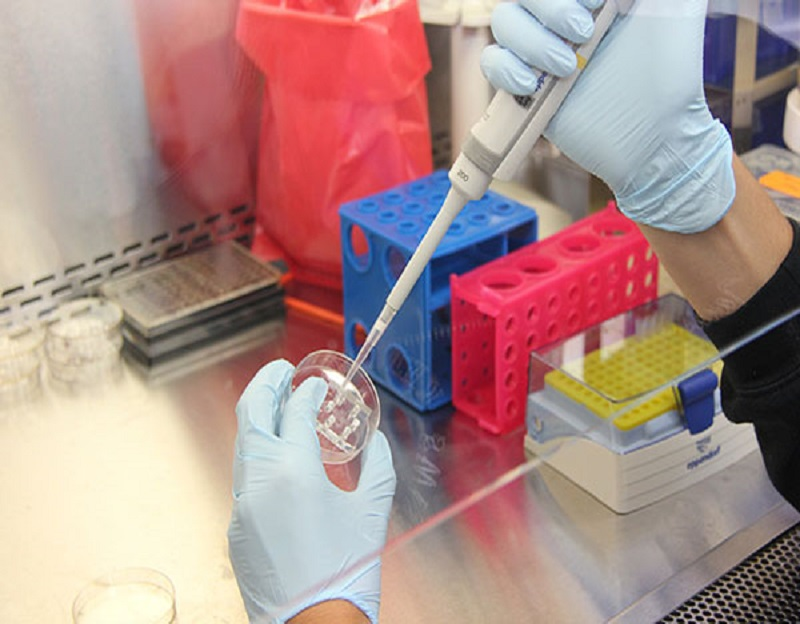# What is a Hypothesis?

Apr 01, 2020A hypothesis is basically a form of proposed explanation for a specific phenomenon. Scientific hypotheses are generally based on previous observations that aren’t generally explained with various scientific theories. Many people use “hypothesis” synonymously with that of “theory” which is actually not right. A scientific hypothesis isn’t the same as a theory because hypothesis is not a universally proved concept whereas the theory is a universally proved concept. There’s also the concept called a “working hypothesis” which is a provisionally accepted hypothesis that’s proposed for further research in a specific field.

## The meaning of the term “hypothesis”

A hypothesis is a theory or an idea that’s not proven. In spite of that, a hypothesis has the potentials of leading into an actual theory, thereby providing more scopes of research and discussions.

## Goals of a hypothesis

The main goal of a hypothesis is to aid in the explanation of the experiment or research. In simpler words, it can be said that a hypothesis will:

• Explain the purpose of the research,
• Identify the variables used in the research.

## Characteristics of a good hypothesis

A hypothesis must:

• Be logical,
• Should use precise language,
• Must be testable with experimentation or research.

## Scientific hypothesis

A scientific hypothesis is often called to be a form of “educated guess”.  That’s because it provides a suggestive solution based on evidence. The term “educated guess” is often debatable and is considered incorrect by many scientists. Experimenters may reject several hypotheses before coming to the actual solution of a problem.

A scientific hypothesis is proposed on the basis of 5 things. These are:

• Testability: A hypothesis is considered to be testable if it’s possible to decide whether it’s true or false in future.
• Parsimony.
• Scope: The application of hypotheses to multiple phenomena.
• Fruitfulness: The future prospect of a hypothesis to depict the fact whether the hypothesis will be fruitful or not.
• Conservatism: It’ll have to be seen whether the hypothesis fits with existing knowledge-systems.

## Working hypothesis

A working hypothesis is a little bit different from that of a normal hypothesis. A working hypothesis is provisionally accepted. It’s hoped that a tenable theory can be attained on further research from the hypothesis. Even if the hypothesis fails in future, a working hypothesis is still expected to produce a tenable theory even from its failure.

A working hypothesis is often used as conceptual framework used in qualitative research.

A working hypothesis is perfectly suitable in applied research. Here, it acts as a form of useful guide to solving various problems that are in a formative phase.

So, do remember that a hypothesis must be testable and should be realistic at the same time. Try to make the hypothesis as simple as possible. A complicated hypothesis generally holds no meaning. Through statistics and analytics, a hypothesis must be verified. And before signing off, we would like to add one last thing that a hypothesis is never proved. But the mindset behind the development of a hypothesis should be such that it will get proved in future. With that statement, we’ll sign off for now. Hope you had a good read.